Square Roots 4
Set 4 (10 Questions)
Students use exponents, powers, and roots, and use exponents in working with fractions
From Mr. Anker Tests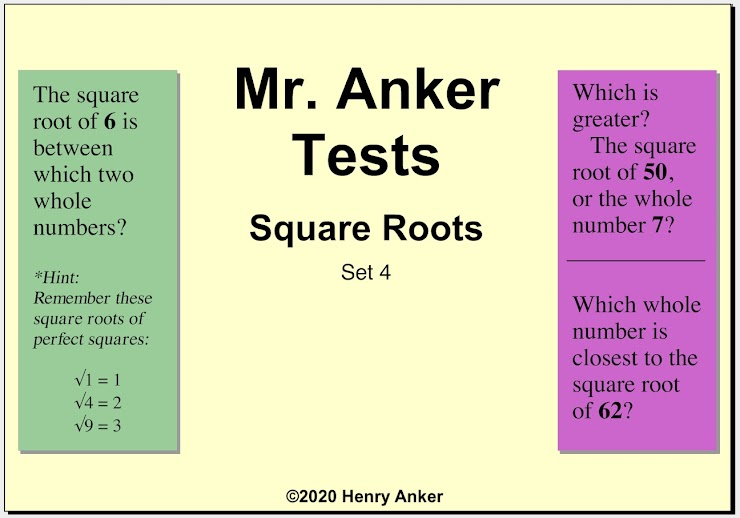Square Roots Help Slide 1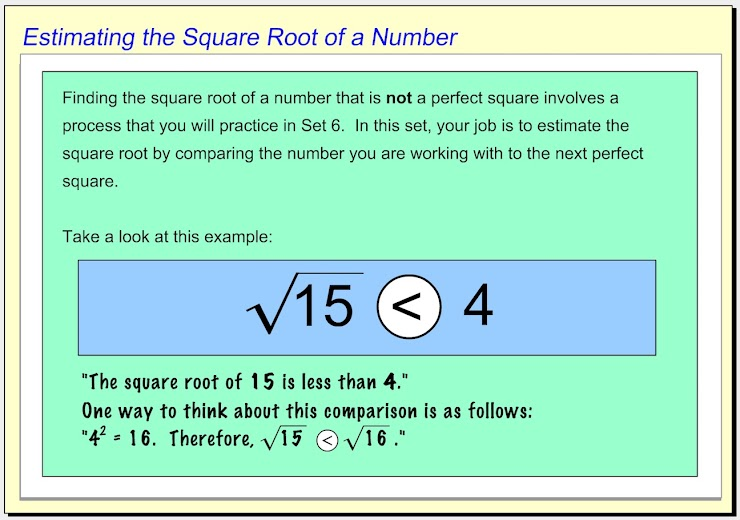Square Roots Help Slide 2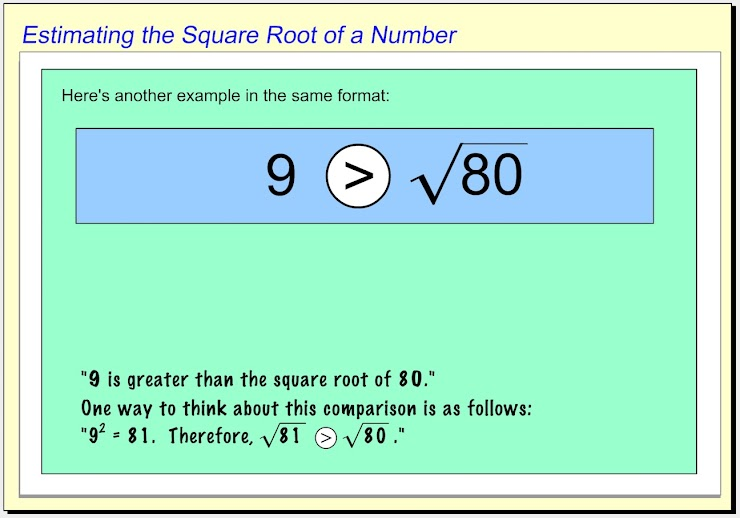Square Roots Help Slide 3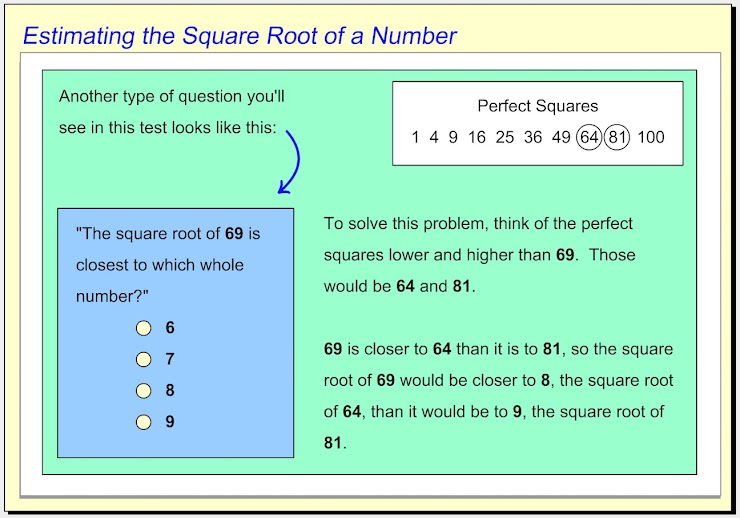Square Roots Help Slide 4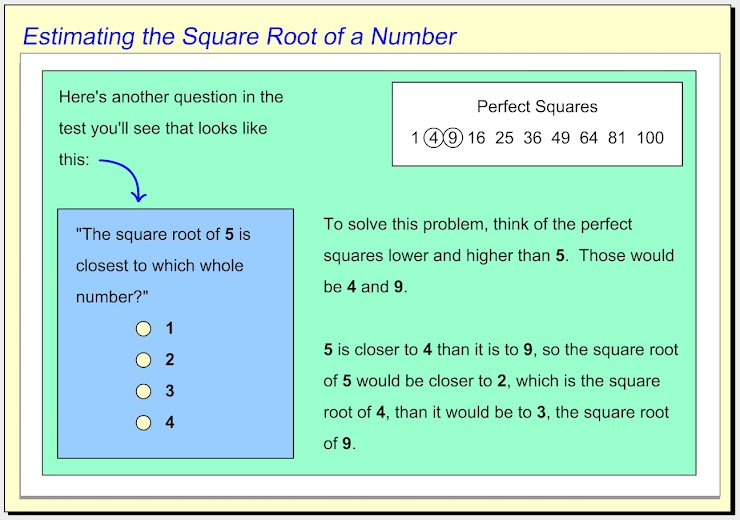1.  The square root of  6  is between which two whole numbers? *
1 point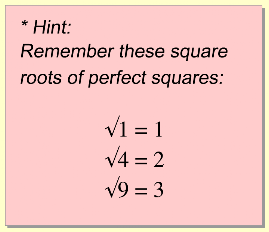2.  The square root of  11  is between which two whole numbers? *
1 point3. Use the dropdown menu below to make this statement true: "Three is __________ the square root of eight." *
1 point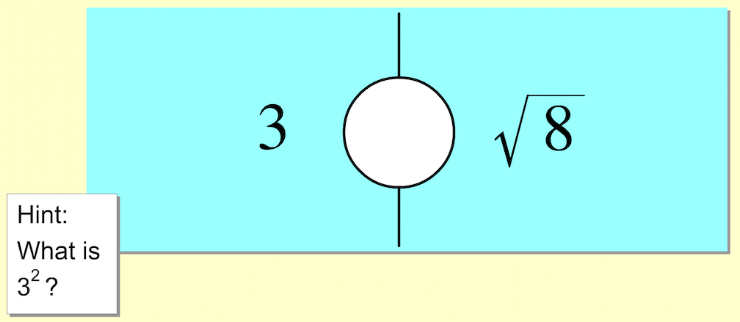4. Use the dropdown menu below to make this statement true: "The square root of seventeen is __________ four." *
1 point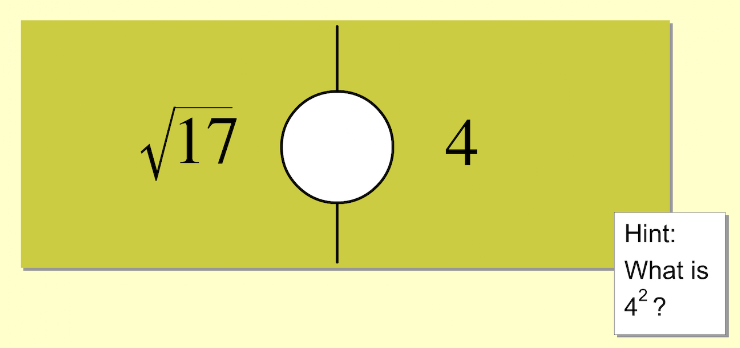5.  The square root of  50  is closest to which whole number> *
1 point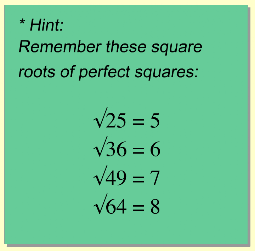6.  Use the dropdown menu below to make this statement true: "The square root of thirty-five is __________ six." *
1 point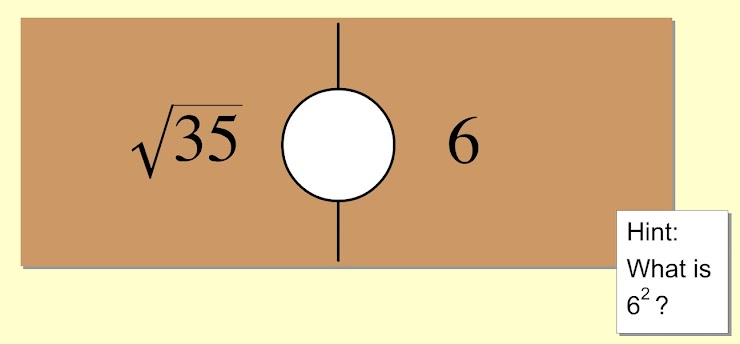7.  The square root of  62  is closest to which whole number? *
1 point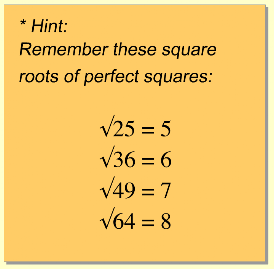8.  Use the dropdown menu below to make this statement true: "The square root of one hundred is __________ ten." *
1 point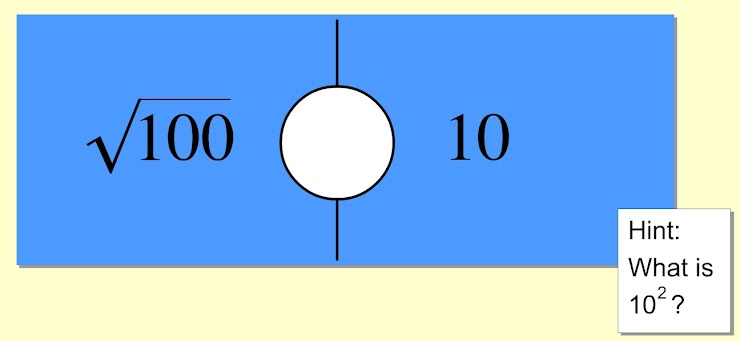9.  Use the dropdown menu below to make this statement true: "The square root of sixty-six is __________ eight." *
1 point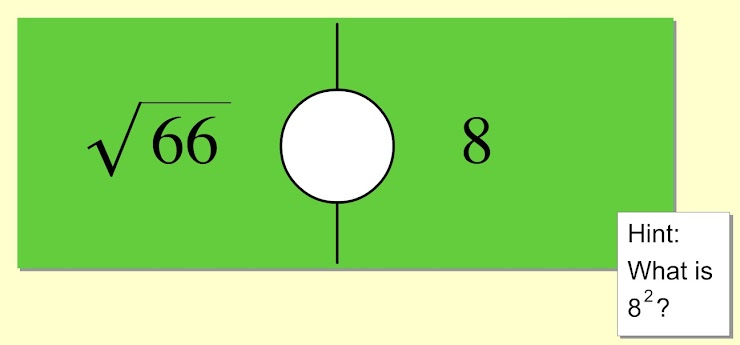10.  Use the dropdown menu below to make this statement true: "The square root of eighty-one is __________ eight." *
1 point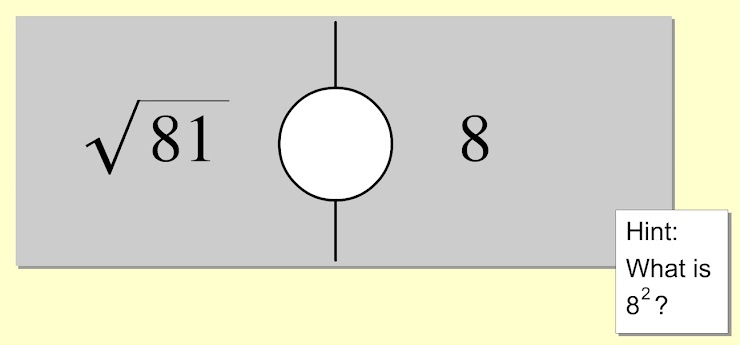Submit
Clear form